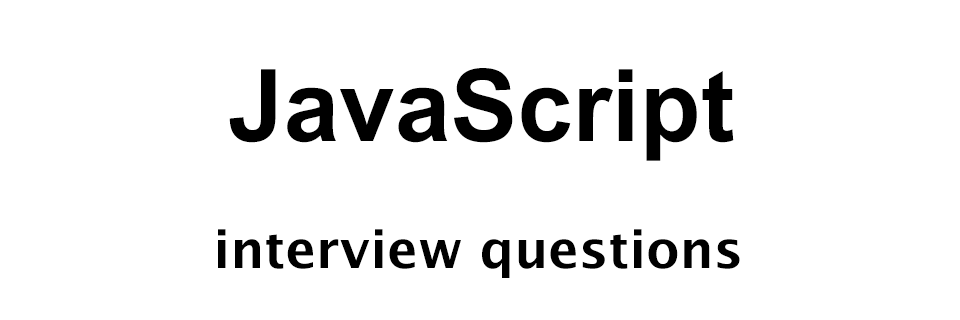# JavaScript Interview Questions - Set 3

JavaScript Interview Questions## Q1: What are the different ways of creating an array in JavaScript?

We can create arrays in JavaScript in the following three ways.

• Array literal
• Instance of Array
• Array constructor

### Array literal

In the following example we are creating an array having three elements using array literal approach.

``````var arr = [1, 2, 3];
``````

### Instance of Array

In the following example we are creating an array having three elements by instantiating Array.

``````var arr = new Array();
arr = 1;
arr = 2;
arr = 3;
``````

### Array constructor

In the following example we are creating an array having three elements using array constructor.

``````var arr = new Array(1, 2, 3);
``````

## Q2: What are the different ways of creating an object in JavaScript?

We can create an object in JavaScript in the following ways.

• Object literal
• Instance of Object
• Object constructor

### Object literal

In the following example we are creating an object having three properties using object literal approach.

``````var obj = {
name: 'Yusuf Shakeel',
score: 10,
};
``````

### Instance of Object

In the following example we are creating an object having three properties by instantiating Object.

``````var obj = new Object();
obj.name = 'Yusuf Shakeel';
obj.score = 10;
``````

### Object constructor

In the following example we are creating an object having three properties using object constructor.

``````var User = function(name, score, username) {
this.name = name;
this.score = score;
};

var obj = new User('Yusuf Shakeel', 10, 'yusufshakeel');
``````

## Q3: What was the original name of JavaScript?

JavaScript was initially named Mocha.

## Q4: What is negative infinity in JavaScript?

Negative infinity is a number we get when we divide negative numbers by zero.

The following code will give us `-Infinity`.

``````console.log(-1/0);
``````

## Q5: What are the pop up boxes in JavaScript?

Following are the pop up boxes in JavaScript.

• Confirm
• Prompt

## Q6: What are alert box and how will you create a simple alert box in JavaScript?

In the following example we are creating an alert box that alerts a message to the user.

``````alert("Welcome back!");
``````

## Q7: What are confirm box and how will you create a simple confirm box in JavaScript?

Confirm box is used to verify or confirm some action by the user.

If the user confirms then confirm box returns `true` otherwise, `false`.

In the following example we will print "Accepted" if the user confirm otherwise, we will print "Rejected".

``````var isConfirmed = confirm('Do you want to proceed?');

if (isConfirmed === true) {
console.log('Accepted');
} else {
console.log('Rejected');
}
``````

## Q8: What are prompt box and how will you create a simple prompt box in JavaScript?

Prompt box is used to get user input.

If user provides some data and clicks the "OK" button then the prompt box returns the submitted data. Otherwise, we get `null`.

In the following example we are creating a simple prompt box.

``````var name = prompt('Enter your name');
console.log(name);
``````

## Q9: What is Hoisting in JavaScript?

Hoisting is a default JavaScript behaviour of moving all the declarations at the top of the current scope (top of the current script or top of the current function).

The following code is an example of hoisting.

``````console.log("Value of x: " + x);
var y = x + 10;
console.log("Added 10 to x and assigned it to y. So y = " + y);

// now declaring x and assigning value
var x = 10;
``````

Output:

``````Value of x: 10
Added 10 to x and assigned it to y. So y = 20
``````

## Q10: What will be the output of the following JavaScript code?

``````function foo() {
console.log(x);
}

var x = 10;

foo();
``````